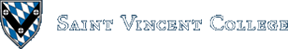Computing & Information Systems    DepartmentSearch CIS Site      Tutorials

## Software Design Using C++

### Introduction

Functions are used to break a programming problem up into reasonable chunks. A function should be cohesive, which means that it should have one overall task. Do not have a function carry out several unrelated actions. When a function is called, the code for the function is executed. When the function code has been executed, program execution continues at the point right after the function call.

Parameters are used to send data into a function. Ordinary parameters use call-by-copy. This means that each formal parameter (parameter name used inside the function code) gets a copy of the value of the corresponding actual parameter (the variable or value that is used in the function call). Of course, the first actual parameter gets matched with the first formal parameter, the second actual parameter with the second formal parameter, etc.

Reference parameters are used to send data back out of a function. A reference parameter is normally indicated by an & between the type name and the formal parameter name. Values can also be sent into the function via a reference parameter. The key idea is that any change made by the function to such a parameter is sent back out of the function. A reference parameter is said to use call-by-reference.

Data can also be send back out of a function in the function name itself. This is accomplished by using a return statement inside the code for the function. For example, `return x;` would send the value of `x` back in the function name.

### A First Example

Let's look at an example program, fn.cpp, that illustrates many of these features. Read through the code and try to identify the ways that data is sent into and back out of the functions. (If you wish, save a copy of the code, compile it, and run it to see more exactly what it does.) Then read the following description to see if you got it right!

### The Run-Time Stack

Program execution begins at the start of the `main` function, of course. The main function in this program has variables `Length`, `Width`, `Area`, and `Perim`. Space is saved for these variables in an area of main memory called the run-time stack. The section of the run-time stack for the `main` function is shown below. Note that there is more room available at the top end of this stack should we need it. Since the `main` function begins by assigning values to variables `Length` and `Width`, these values are shown in the drawing of the run-time stack.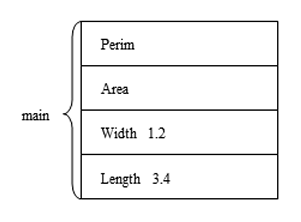Next, the `main` function calls the `FindArea` function as follows:

`FindArea(Length, Width, Area);`

The variables `Length`, `Width`, and `Area` inside these parentheses are the actual parameters. They correspond in order from left to right to the three formal parameters found in the code for the function, shown below.

 `````` void FindArea(float Length, float Width, float & Area) { Area = Length * Width; } ``````

When a function call is reached, a stack frame (sometimes called an activation record) is placed on the run-time stack. We will use a simplified picture of a stack frame that ignores some of the technical details (such as the local base or base pointer) and does not look at differences that exist between various platforms (such as Windows versus Linux).

In our picture, the first items in the stack frame are the parameters. Then comes the so-called return address, while the last items would be local variables (but our example has no local variables). The first two formal parameters are non-reference (call-by-copy) parameters. Thus, in the picture of the run-time stack below, you see that the 3.4 and 1.2 have been copied into these formal parameters. The third formal parameter, `Area`, is a reference parameter. No value is stored in a reference parameter. Rather, it contains a pointer to the corresponding actual parameter. In our example the formal parameter `Area` contains a pointer to the variable also called `Area` in the `main` function section of the stack. The pointer is essentially the main memory address of the item pointed to, but is more conveniently drawn as an arrow instead of a number.

The return address is the location in the compiled, executable code to return to when the called function has completed. In our example, program execution should continue on the line following the function call. Since this return address is a number indicating a location in the compiled machine code, no attempt is made to show the number itself, as it will vary from computer to computer and may even vary from one run to another on the same computer.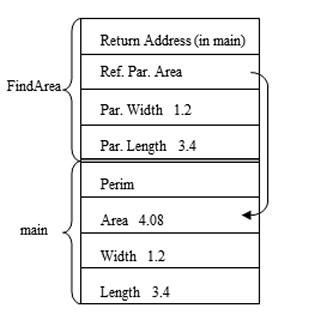Note that the stack grows from high addresses to low addresses in memory, at least on most computers. This is backwards of what you might expect, but works fine as long as you don't run out of free memory by running up against something else at the low end of memory. However, even on a computer whose stack grows from low to high memory addresses, it is possible to run up against something else on the high end.

When the function multiplies the length and width to get the area, this value, 4.08, is stored by following the pointer for the `Area` reference parameter. Thus the answer gets stored in the `Area` variable for function `main`. This is the mechanism that a reference parameter uses in order to send an answer back to whatever function called this function.

When the end of the function `FindArea` is reached, the return address is used to tell where to continue with the execution of the program. The stack frame for `FindArea` is also removed from the stack. (Actually, it is not really erased. Rather, some indicator of where the top item is on the stack is just moved. This indicator is typically called the stack pointer.) Note that the parameters `Length`, `Width`, and `Area` are now gone. They have a short lifetime! Their lifetime begins when the function is called and is over when the function is finished.

One can also talk about the scope of a variable, parameter, or other identifier. The scope is that section of code where it is legal to use that identifier. In our example program, parameters `Length`, `Width`, and `Area` have as scope the section of code comprising function `FindArea`. Those parameters don't exist outside of this function.

In any case, once function `FindArea` ends and its stack frame is removed, the run-time stack looks like this: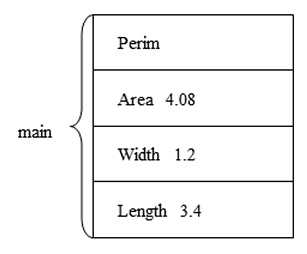Next, as program execution continues in the `main` function, we see that `main` calls function `FindPerim` as follows:

 `````` Perim = FindPerimeter(Length, Width); ``````

Since this function is returning a value in its function name, this may well be handled by using a mechanism similar to a reference parameter. We will assume that the compiler will set things up so that a temporary variable is put on the stack for the `main` function before putting on the stack frame for function `FindPerimeter`. The stack frame for `FindPerimeter` will start with the parameters (both value or non-reference parameters) `Length` and `Width`, each containing a copy of the corresponding actual parameter. Next, in order to return a value via the function name, the stack frame has a location (labelled `Temp` in the drawing below) that acts like a reference parameter in that it contains a pointer to the `Temp` variable for the `main` function. Finally we have the return address of where to go back to in the `main` function. There are no local variables for this function, but if there were, they would after the return address.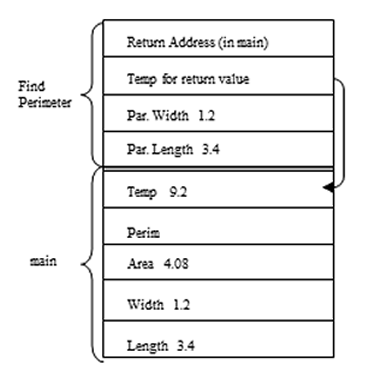Function `FindPerimeter` executes a return statement in order to send a value back in the function name:

 `````` return 2 * (Length + Width); ``````

This returns 9.6 by storing it in the `Temp` location pointed to by our `Temp` parameter. Thus the 9.6 gets stored in the area for function `main`. The return address is used to send program control back to the correct spot in function `main`, and the stack frame for `FindPerimeter` is removed. Back in function `main`, the 9.6 in `Temp` is copied into the variable `Perim`, thanks to the assignment statement quoted above the last stack drawing. The `Temp` variable is then removed from the stack as it is no longer needed. (Note that a smart compiler might be able to eliminate this `Temp` variable and just arrange things so that the pointer from the stack frame points right to variable `Perim`.)

Function `main` then arranges to call `FindArea` a second time. The only difference is that this time literal numbers, not variables, are used as the first two actual parameters. This can be done when the corresponding actual parameters are non-reference parameters, but cannot be used when we have a reference parameter. Why? Well, remember that a reference parameter lets us change the corresponding actual parameter. There would be no way to change a literal, since it is not a variable!

The `main` function then calls function `FindPerimeter` a second time. This time the function call is embedded inside of an output statement. In simplified form, the function call looks like this:

 `````` cout << FindPerimeter(5.67, 2.5); ``````

This would be set up much like the first call of `FindPerimeter`. A `Temp` variable would be put on the stack for function `main`, and the stack frame for `FindPerimeter` would contain a pointer back to this `Temp` variable. The answer returned is sent back via this pointer and stored in the `Temp` variable. Then the value in `Temp` is used in the output statement. After that the `Temp` variable is removed from the stack. That is essentially the end of the program, so the rest of the variables for `main` also are removed.

### Another Example

Let's look at another example, params.cpp, which does nothing useful other than to illustrate parameter passing. Read through the code for this one, and then check the pictures of the run-time stack shown below. The first picture shows that the run-time stack starts out by reserving space for any global variables. A global variable is one whose scope is the entire source code file. You create a global variable by declaring it outside of any function, usually toward the top of your file. Global variables are usually considered to be harmful and should be avoided by beginning programmers. It is shown here only to show how it might operate. (On many computers, global variables and global constants are stored in a different region of memory, not in the run-time stack region. For our purposes it makes no difference.)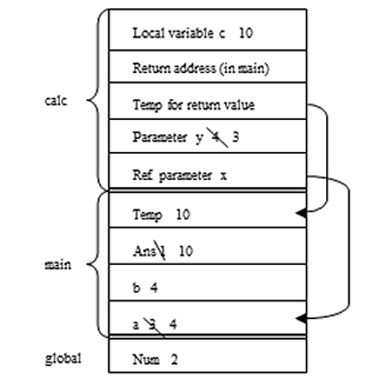The preceding picture of the run-time stack also shows the variables for function `main` as well as the stack frame for function `calc`. Note that the first parameter of `calc` is a reference parameter (using a pointer), but the second parameter is not (and so gets a copy of the actual parameter). Since `calc` is designed to send a value back via the function name, we once again use a `Temp` variable with a pointer to it in the stack frame. Also note that function `calc` contains a local variable `c`, which is shown in the stack frame after the return address.

The computations in function `calc` are rather arbitrary. First, the `++` operator is used to increment `x`. Since `x` is a reference parameter, one follows the pointer so that `a` is incremented from 3 to 4. Similarly, the `--` operator is used to decrement `y`. Since `y` is a value parameter, not a reference parameter, it contains a value, 4, which gets decremented to 3. The value of `x + 2 * y` is then assigned into local variable `c`. Since this works out to be 10, the value 10 gets assigned into `c`. Next `calc` outputs the values of `x`, `y`, `c`, and `num` so that one can see what they are. Of course, we should get 4, 3, 10, and 2. Note that the global variable `num` is accessible throughout all of the functions of our program. In other words, its scope is the whole program file. In contrast, `x`, `y`, and `c` have just the section of code of function `calc` as their scope.

Finally, `calc` returns the value of `c` in the function name. Thus 10 is copied into the `Temp` variable in the area for the `main` function (via the pointer, of course). The return address sends program execution back to the `main` function, and the stack frame is removed. Once the 10 in `Temp` has been copied into `ans`, `Temp` is also removed from the stack.

Next, the `main` function prints the values of `a`, `b`, `ans`, and `num`. Of course, we get 4, 4, 10, and 2. At this point the `compute` function is called. This function has no return value and has just one parameter, which is a value parameter and hence uses "call by copy", not "call by reference". This leads to the following picture of the run-time stack: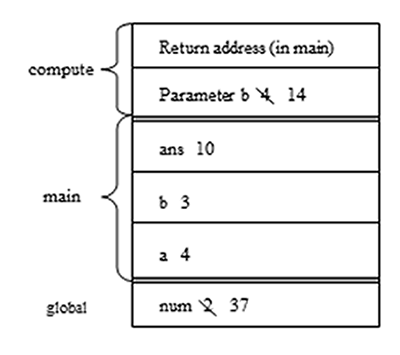Compute adds 10 to the parameter `b` and changes the global variable `num` to 37. Although this is one way to send an answer back out of a function, it is poor programming practice in nearly all cases. When one calls a function, one expects that answers are sent back in the function name and/or via reference parameters. Once does not expect that the function has a "hidden agenda" of changing a global variable. Changing global variables within functions makes it very difficult to write and maintain a large program. You are strongly advised not to use this capability.

Of course, function `compute` also prints some values, namely 14 and 37. Then the return address is used to send control back to the `main` function. The stack frame for `compute` is then removed from the stack. The `main` function then prints the values of `a`, `b`, `ans`, and `num`. This time the results are 4, 4, 10, and 37. That is the end of the program.

### A Final Example

Let's look at another nonsense example, params2.cpp. This program also contains a global variable, `b`. Recall that the use of global variables is usually a very bad idea. Here one is used just to illustrate a point.

The `main` function begins by initializing the global variable and `main`'s own variable `ans`. Then it calls function `compute`. Note that `compute` has one parameter, a reference parameter. There is also a local variable, `b`. This leads to the following picture of the run-time stack: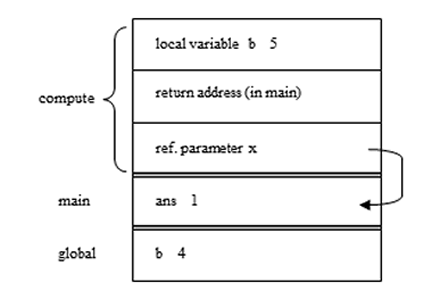Note that we have two variables named `b`. This can be confusing. For ordinary variables this is resolved by saying that the variable with the most local scope is the one meant. Thus, inside of function `compute`, any variable `b` means the `b` local to this function, not the global `b`. In function `main`, of course, any reference to `b` must mean the global one, since that is the only one whose scope includes `main`.

As `compute` runs, it looks up `x` (by following the pointer and getting 1), adds 4, and stores the result in `b`, which means the local `b` as was just said. The program then prints the values of `x` and `b`, which must be 1 and 5.

Function `compute` then calls function `calc`. So we must put another stack frame onto the run-time stack. Since `calc` returns a value in the function name we will again place a `Temp` variable on the stack to hold the value returned and then add the stack frame for `calc`, with a pointer to this new `Temp` variable in the area for function `compute`. The stack thus looks like the following: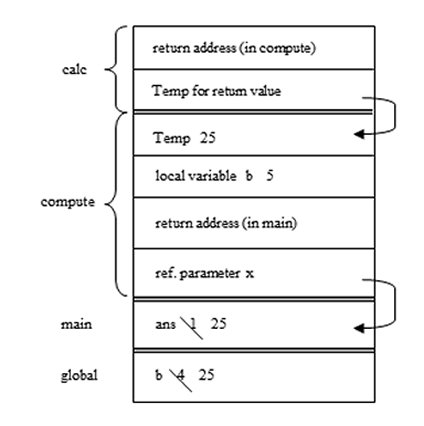Function `calc` begins by placing 25 into `b`. Which `b` is that? From the rules above it must mean the global `b`. This is called "static scoping", meaning that we can tell which variable is meant just by the position we are at on the page of code. Note, however, that there are languages which use dynamic scope rules. In this situation, such a language would conclude that the the variable meant was the `b` local to function `compute` since that is the function from which `calc` was called! In such a language, the variable that is meant depends on the context of function call.

In our case, function `calc` goes on to print the global `b`. Of course, 25 is printed. It then returns the 25 via the function name. In reality, we follow the `Temp` pointer and store the 25 in the `Temp` variable in the stack frame for function `compute`. The return address sends us back to function `compute`, and `calc`'s stack frame is removed.

Upon return to function `compute` we find ourselves in the middle of the assignment statement `x = calc()`. The value of the right hand side has been figured out and is sitting in `Temp`. It would look like we should just copy the 25 from `Temp` into the variable `x`, but wait! We see that `x` is a reference parameter. Thus we follow the pointer for the reference parameter and copy the 25 into the variable `ans` in the `main` function. The `Temp` variable in function `compute` is probably removed from the stack as soon as we are finished with it. Next, `compute` prints the values of `x` and `b`, which of course are now 25 and 5. (Note that `b` is 5 since `b` refers to the local `b`, not the global one.) Function `compute` now ends. We are returned to the `main` function, `compute`'s stack frame is removed, and `main` ends by printing the values of `ans` and `b`, which are now 25 and 25.

### Related Items

 Simple FunctionsIntroductory Topic. More Complex FunctionsIntroductory Topic.
Back to the main page for Software Design Using C++

Author: Br. David Carlson with contributions by Br. Isidore Minerd
Last updated: September 20, 2016
Disclaimer